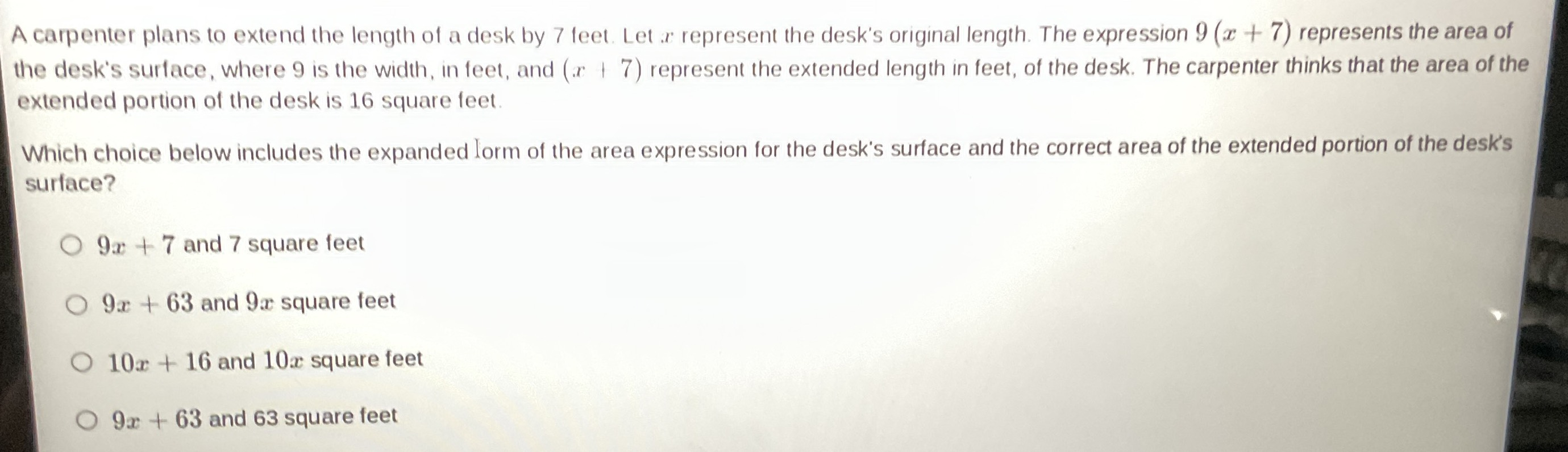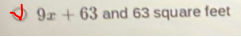### ¿Todavía tienes preguntas de matemáticas?

Pregunte a nuestros tutores expertos
Algebra
PreguntaA carpenter plans to extend the length of a desk by $$7$$ teet. Let $$x$$ represent the desk's original length. The expression $$9 ( x + 7 )$$ represents the area of the desk's surface, where $$9$$ is the width, in feet, and $$( x + 7 )$$ represent the extended length in feet, of the desk. The carpenter thinks that the area of the extended portion of the desk is $$16$$ square leet. Which choice below includes the expanded lorm of the area expression for the desk's surface and the correct area of the extended portion of the desk's surface?

$$9 x + 7$$ and $$7$$ square feet

$$9 x + 63$$ and $$9 x$$ square feet

$$10 x + 16$$ and $$10 x$$ square feet

$$9 x + 63$$ and $$63$$ square feet